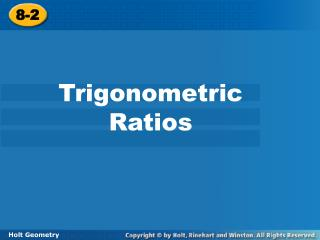DownloadDownload Presentation8-2

# 8-2

Télécharger la présentation## 8-2

- - - - - - - - - - - - - - - - - - - - - - - - - - - E N D - - - - - - - - - - - - - - - - - - - - - - - - - - -
##### Presentation Transcript

1. 8-2 Trigonometric Ratios Holt Geometry

2. Objectives Find the sine, cosine, and tangent of an acute angle. Use trigonometric ratios to find side lengths in right triangles and to solve real-world problems.

3. The Legend of SOHCAHTOA

4. The sons of the squaw on the hide of the hippopotamus is equal to the sons of the squaws on the other two hides.

5. A trigonometric ratio is a ratio of two sides of a right triangle. • Sine • Cosine • Tangent

6. SOH CAH TOA Sin A = Cos A = Tan A =

7. Example 1A: Finding Trigonometric Ratios Write the trigonometric ratio as a fraction and as a decimal rounded to the nearest hundredth. sin J cos J tan K

8. Write the trigonometric ratio as a fraction and as a decimal rounded to the nearest hundredth. cos A tan B sin B

9. Example 2: Finding Trigonometric Ratios in Special Right Triangles Use a special right triangle to write cos 30° as a fraction.

10. s 45° 45° s Use a special right triangle to write tan 45° as a fraction.

11. Caution! Be sure your calculator is in degree mode, not radian mode. Example 3A: Calculating Trigonometric Ratios Use your calculator to find the trigonometric ratio. Round to the nearest hundredth. sin 52° sin 52°  0.79

12. Example 3B: Calculating Trigonometric Ratios Use your calculator to find the trigonometric ratio. Round to the nearest hundredth. cos 19° cos 19°  0.95

13. Example 3C: Calculating Trigonometric Ratios Use your calculator to find the trigonometric ratio. Round to the nearest hundredth. tan 65° tan 65°  2.14

14. Example 4A: Using Trigonometric Ratios to Find Lengths Find the length. Round to the nearest hundredth. BC

15. Caution! Do not round until the final step of your answer. Use the values of the trigonometric ratios provided by your calculator.

16. Example 4B: Using Trigonometric Ratios to Find Lengths Find the length. Round to the nearest hundredth. QR

17. Example 4C: Using Trigonometric Ratios to Find Lengths Find the length. Round to the nearest hundredth. FD

18. Check It Out! Example 4a Find the length. Round to the nearest hundredth. DF

19. Check It Out! Example 4a Continued Write a trigonometric ratio. Substitute the given values. Multiply both sides by DF and divide by sin 51°. DF 21.87 cm Simplify the expression.

20. Check It Out! Example 4c Find the length. Round to the nearest hundredth. BC

21. Check It Out! Example 4c Continued Write a trigonometric ratio. Substitute the given values. Multiply both sides by BC and divide by tan 18°. Simplify the expression. BC 36.93 ft

22. Example 5: Problem-Solving Application The Pilatusbahn in Switzerland is the world’s steepest cog railway. Its steepest section makes an angle of about 25.6º with the horizontal and rises about 0.9 km. To the nearest hundredth of a kilometer, how long is this section of the railway track?

23. 1 Understand the Problem 0.9 km Example 5 Continued

24. Use a special right triangle to write each trigonometric ratio as a fraction. 1. sin 60° 2. cos 45°

25. Homework Page 529: 22-42 Even